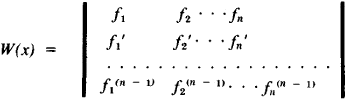# Wronskian

Also found in: Wikipedia.

## Wronskian

[′vrän·skē·ən]
(mathematics)
An n × n matrix whose i th row is a list of the (i - 1)st derivatives of a set of functions f1, …, fn ; ordinarily used to determine linear independence of solutions of linear homogeneous differential equations.
McGraw-Hill Dictionary of Scientific & Technical Terms, 6E, Copyright © 2003 by The McGraw-Hill Companies, Inc.
The following article is from The Great Soviet Encyclopedia (1979). It might be outdated or ideologically biased.

## Wronskian

a functional determinant composed of n functions f1(x), f2(x)....,fn(x) and their derivatives up to the order n - 1 inclusive:The vanishment of the Wrońskian [W(x) = 0] is a necessary and, under certain additional assumptions, a sufficient condition for the linear dependence between the given n functions, differentiated n - 1 times. Based on this, the Wrońskian is used in the theory of linear differential equations. The Wrońskian was introduced by J. Wroński in 1812.

The Great Soviet Encyclopedia, 3rd Edition (1970-1979). © 2010 The Gale Group, Inc. All rights reserved.
References in periodicals archive ?
It is well-known that f is non-degenerate over C if and only if the Wronskian W = W([f.sub.1], ..., [f.sub.n+1]) of [f.sub.1], ..., [f.sub.n+1] is not identically equal to zero.
In the literature, DWPs have been studied by using various techniques such as the WKB approximation [33, 34], asymptotic iteration method (AIM) , and the Wronskian method .
Following , we introduce the Wronskian of two sequences [{[[psi].sub.1]}.sub.j[member of]N], and [{[[psi].sub.2]}.sub.j[member of]N],
Many methods have been developed to find the explicit solutions of nonlinear evolution equations; example of such methods are the first integral method , Jacobi elliptic function method , Hirota bilinear method , Wronskian determinant technique , F-expansion method , Darboux Transformations , Backlund transformation method , Miura transformation , homotopy perturbation method , and Adomian decomposition method .
hence [[g.sub.2], [y.sub.m]] = 0, and by the Wronskian identity, we get
where W[[phi](x, [lambda]), [psi](x, [lambda])] is Wronskian of the vector solutions [phi](x, [lambda]) and [psi](x, [lambda]).
 constructed its Wronskian and Grammian solutions.
Let f and g be a-differentiable; the fractional Wronskian function is defined by
Let W(f g; x) = f(x) g'(x) - f'(x)g(x) denote the Wronskian of functions f(x) and g(x).
and [W.sub.n] is the Wronskian of Whittaker functions 

Site: Follow: Share:
Open / Close